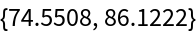#Function Repository Resource:

# BootstrapStatistics

Bootstrap a single statistic or a list of statistics

Contributed by: Katja Della Libera
 ResourceFunction["BootstrapStatistics"][data,n] returns a list of n bootstrapped datasets. ResourceFunction["BootstrapStatistics"][data,n,func] returns a list of the values of func applied to n bootstrapped datasets. ResourceFunction["BootstrapStatistics"][data,n,funclist] returns a list each function in funclist applied to n bootstrapped datasets.

## Details and Options

Bootstrapping is a statistical method that resamples data with replacement to asses measures of accuracy

## Examples

### Basic Examples (2)

Create five bootstrapped datasets by resampling from an original dataset:

 In:=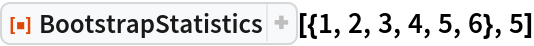Out=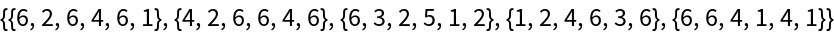Compute the Mean of a dataset:

 In:=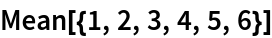Out=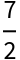Compare it to the means of ten bootstrapped samples:

 In:=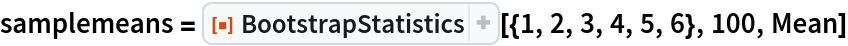Out=In:=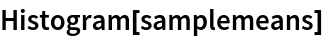Out=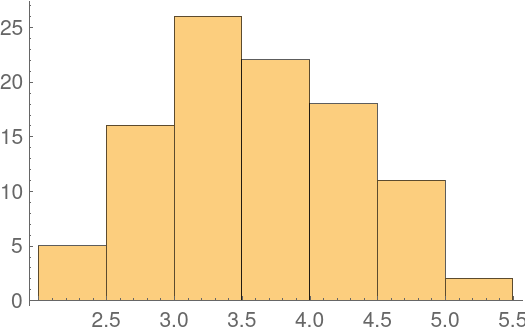### Scope (1)

Pass a list of functions to BootstrapStatistics to get a list of lists of results:

 In:=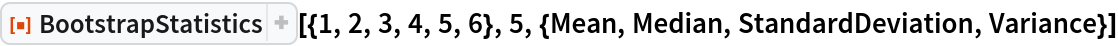Out=### Applications (4)

The example dataset "BuffaloSnow" shows snowfall records for Buffalo, New York:

 In:=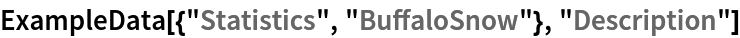Out=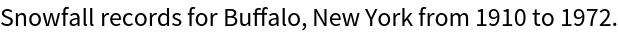With traditional methods, we can only get one estimate for the mean snowfall:

 In:=Out=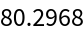Using the bootstrap method, we can get a larger set giving us a better idea of how much this measure varies:

 In:=Out=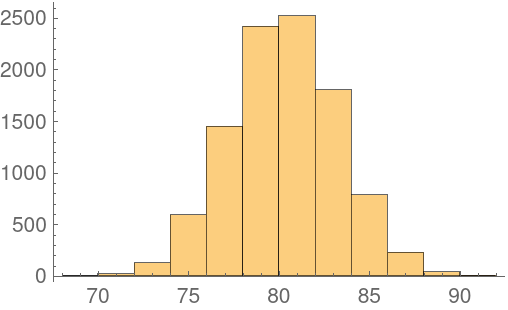We can use Quantile to find the 95% confidence interval:

 In:=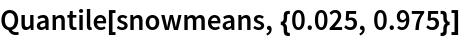Out=